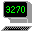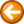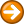Round - round a number\$return = round(number[,precision][,TRIM])

Where:

 \$return the rounded number.
 number is a decimal number.
 precision is the number of decimal places to include in the rounded result. If the precision specified is greater than the number of digits in the input, then trailing zeros will be added to the output.
 TRIM trims trailing zeros and trailing decimal point if nothing follows it after trimming zeros.

number and precision may be variables.

The Round command rounds a number to the specified precision.

Examples

\$result = round("3.1415",2)

Sample Script

' round pi

\$pi = 3.1415

\$a = round(\$pi)

\$b = round(\$pi,1)

\$c = round(\$pi,10)

MsgBox(\$a & \$CRLF & \$b & \$CRLF & \$c & \$CRLF)

exit